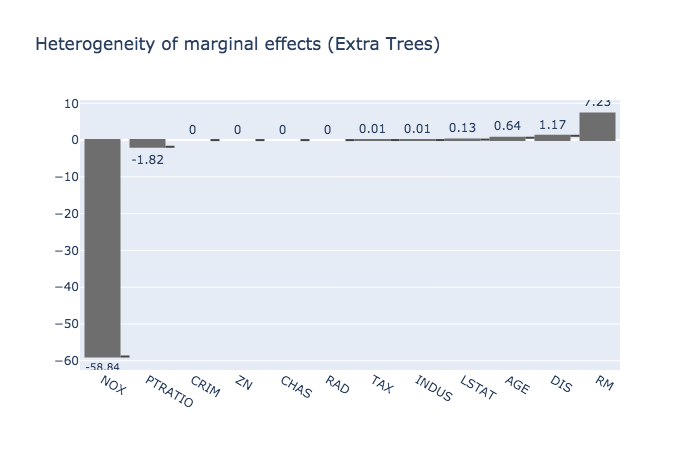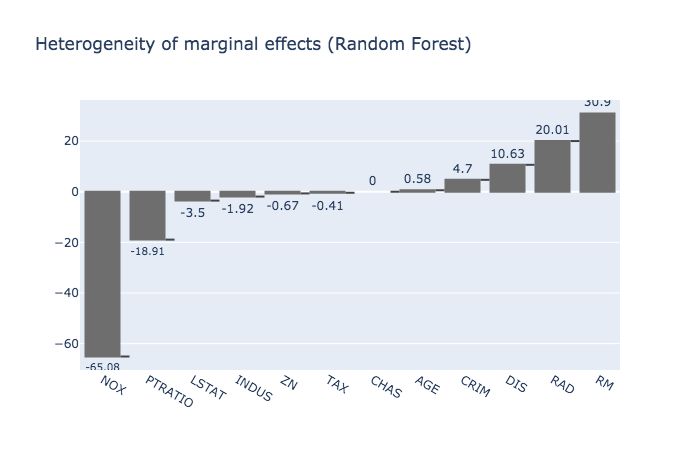As mentioned here 2 weeks ago, when introducting the teller, there is an increasing need for transparency and fairness in Statistical/Machine Learning (ML) models predictions. Some ML models which are not linear are considered as being black boxes, but they may exhibit high accuracy numbers. Since we do not want to sacrifice this high accuracy to explainability, we can use the teller, a model-agnostic tool for ML explainability, to understand them a little bit more.

In this post, we are going to use the teller to compare two ML models on the Boston Housing dataset:

Why am I using this dataset this much? Not because I’m a real estate agent, but:

• It’s widely used and well-studied in ML
• It’s directly available to everyone through (the also widely used) R package MASS, and Python’s scikit-learn
• It has a moderate size, ideal for demos
• It allows to derive insights which are interesting, and understandable by almost everyone

I also found the paper: Harrison, D. and Rubinfeld, D.L. (1978) Hedonic prices and the demand for clean air. J. Environ. Economics and Management 5, 81–102, to be an interesting and accessible read. If you are interested.

Data description: The response (variable to be explained) is MEDV, Median value of owner-occupied homes in $1000’s. • CRIM per capita crime rate by town • ZN proportion of residential land zoned for lots over 25,000 sq.ft. • INDUS proportion of non-retail business acres per town • CHAS Charles River dummy variable (= 1 if tract bounds river; 0 otherwise) • NOX nitric oxides concentration (parts per 10 million) • RM average number of rooms per dwelling • AGE proportion of owner-occupied units built prior to 1940 • DIS weighted distances to five Boston employment centres • RAD index of accessibility to radial highways • TAX full-value property-tax rate per$10,000
• PTRATIO pupil-teacher ratio by town
• LSTAT % lower status of the population
• MEDV Median value of owner-occupied homes in $1000’s (the reponse) ### Install the package and import data Currently, the teller’s development version can be obtained from Github as: !pip install git+https://github.com/Techtonique/teller.git  Packages and dataset: # Import packages and data import teller as tr import pandas as pd import numpy as np import lightgbm as lgb import xgboost as xgb import math from sklearn import datasets, linear_model from sklearn.ensemble import RandomForestRegressor, ExtraTreesRegressor, BaggingRegressor from sklearn import datasets from sklearn.model_selection import train_test_split, GridSearchCV from sklearn import metrics # import data boston = datasets.load_boston() X = np.delete(boston.data, 11, 1) y = boston.target col_names = np.append(np.delete(boston.feature_names, 11), 'MEDV')  ### Model training and explanations We split data into a training and a testing set: X_train, X_test, y_train, y_test = train_test_split(X, y, test_size=0.2, random_state=123) print(X_train.shape) print(X_test.shape) print("mean of y_test: ") print(np.mean(y_test)) print("std. deviation of y_test: ") print(np.std(y_test))  Now we train our 2 models, starting with the Extremely Randomized Trees: # fit an Extra Trees model to Boston Housing data regr2 = ExtraTreesRegressor(n_estimators=1000, max_features=int(math.sqrt(X_train.shape)), random_state=123) regr2.fit(X_train, y_train) # creating the explainer expr2 = tr.Explainer(obj=regr2) # fitting the explainer (for heterogeneity of effects only) expr2.fit(X_test, y_test, X_names=col_names[:-1], y_name=col_names[-1], method="avg") # confidence intervals and tests on marginal effects (Jackknife) expr2.fit(X_test, y_test, X_names=col_names[:-1], y_name=col_names[-1], method="ci") # summary of results for the model print(expr2.summary())  Score (rmse): 10.813 Residuals: Min 1Q Median 3Q Max -11.7904 -1.84795 -0.288655 0.937975 18.51445 Tests on marginal effects (Jackknife): Estimate Std. Error 95% lbound 95% ubound Pr(>|t|) NOX -59.4205 2.22045e-16 -59.4205 -59.4205 0 *** PTRATIO -2.00072 0.390455 -2.77528 -1.22616 1.44031e-06 *** CRIM 0 2.22045e-16 -4.40477e-16 4.40477e-16 1 - ZN 0 2.22045e-16 -4.40477e-16 4.40477e-16 1 - CHAS 0 2.22045e-16 -4.40477e-16 4.40477e-16 1 - RAD 0 2.22045e-16 -4.40477e-16 4.40477e-16 1 - TAX 0.0121302 2.22045e-16 0.0121302 0.0121302 0 *** INDUS 0.0125259 3.31241e-16 0.0125259 0.0125259 0 *** LSTAT 0.127336 0.27273 -0.413686 0.668359 0.64158 - AGE 0.643206 6.69456e-15 0.643206 0.643206 0 *** DIS 1.17726 2.45467e-14 1.17726 1.17726 0 *** RM 7.29791 0.201907 6.89738 7.69844 1.37027e-59 *** Signif. codes: 0 ‘***’ 0.001 ‘**’ 0.01 ‘*’ 0.05 ‘.’ 0.1 ‘-’ 1 Multiple R-squared: 0.869, Adjusted R-squared: 0.852 Heterogeneity of marginal effects: mean std min max NOX -58.837937 476.247650 -4564.951708 755.791176 PTRATIO -1.818289 19.700286 -130.395492 38.014987 CRIM 0.000000 0.000000 0.000000 0.000000 ZN 0.000000 0.000000 0.000000 0.000000 CHAS 0.000000 0.000000 0.000000 0.000000 RAD 0.000000 0.000000 0.000000 0.000000 TAX 0.012011 0.102841 0.000000 1.020264 INDUS 0.012403 0.125266 0.000000 1.265120 LSTAT 0.128762 2.955437 -8.391279 27.818439 AGE 0.636900 4.638587 -1.521252 34.266627 DIS 1.165723 14.435157 -24.558015 143.461713 RM 7.228340 28.794942 0.000000 215.621069 NoneExtra Trees predictions for home value are highly sensisitive to air pollution. And increase of 1 in nitrogen oxides concentration (parts per 10 million) leads, all else held constant and on average, to a decrease of 58k$ in median homes’ values. The increase in home value is driven by the number of rooms. We can also note that variables such as criminality rate and the accessibility to radial highways, seem to have a negligible impact on model predictions.

Now, we’ll train a Random Forest on the same dataset, and see what it tells us about its predictions:

# fit a random forest model
regr1 = RandomForestRegressor(n_estimators=1000,
max_features=int(math.sqrt(X_train.shape)),
random_state=123)
regr1.fit(X_train, y_train)

# creating the explainer
expr1 = tr.Explainer(obj=regr1)

# fitting the explainer (for heterogeneity of effects only)
expr1.fit(X_test, y_test, X_names=col_names[:-1], y_name=col_names[-1], method="avg")

# confidence intervals and tests on marginal effects (Jackknife)
expr1.fit(X_test, y_test, X_names=col_names[:-1], y_name=col_names[-1], method="ci")

# summary of results for the model
print(expr1.summary())

Score (rmse):
13.639

Residuals:
Min     1Q  Median       3Q      Max
-10.6667 -1.396 -0.5047  1.25705  22.4512

Tests on marginal effects (Jackknife):
Estimate   Std. Error   95% lbound   95% ubound     Pr(>|t|)
NOX      -65.9852      23.5248     -112.652     -19.3183   0.00603773   **
PTRATIO  -19.0443      5.74131     -30.4335      -7.6551   0.00126512   **
LSTAT      -2.972      3.11832     -9.15791      3.21392     0.342827    -
INDUS    -1.90767      2.88467     -7.63009      3.81474     0.509917    -
ZN      -0.670289     0.429838     -1.52297     0.182394      0.12203    -
TAX     -0.412312    0.0252358    -0.462373    -0.362251  4.10351e-30  ***
CHAS            0  2.22045e-16 -4.40477e-16  4.40477e-16            1    -
AGE      0.583416   5.5788e-15     0.583416     0.583416            0  ***
CRIM      4.74938  1.16039e-13      4.74938      4.74938            0  ***
DIS       10.7329  2.14226e-13      10.7329      10.7329            0  ***
RAD       20.1803      4.93784       10.385      29.9757  8.78367e-05  ***
RM        31.1946     0.809636      29.5885      32.8007  3.33135e-62  ***

Signif. codes:  0 ‘***’ 0.001 ‘**’ 0.01 ‘*’ 0.05 ‘.’ 0.1 ‘-’ 1

Multiple R-squared:  0.835,	Adjusted R-squared:  0.813

Heterogeneity of marginal effects:
mean         std          min          max
NOX     -65.077408  837.110491 -7304.767586  2092.276204
PTRATIO -18.914731   81.475430  -585.195412    40.313679
LSTAT    -3.504697   51.609150  -260.521317   226.189605
INDUS    -1.917253   76.799856  -342.638186   617.598139
ZN       -0.667706    9.494545   -49.129261    41.635391
TAX      -0.408517    3.248658   -32.287535     0.880749
CHAS      0.000000    0.000000     0.000000     0.000000
AGE       0.577696   12.232191   -71.283614    85.560655
CRIM      4.702817   47.496127     0.000000   479.687370
DIS      10.627708   62.112145     0.000000   540.229275
RM       30.895241  169.441083  -247.545527  1346.559346
NoneFor this model too, air pollution is an important variable driving the decrease in home value. The lack of teachers for each kid plays a more important role here, but contrary to Extra Trees, Random Forests give much more importance to the accessibility of radial highways.

### Comparing models

We can finally compare both models side by side, using the teller’s Comparator:

# create object for model comparison
# expr1 is for Random Forest
# expr2 is for Extra Trees
cpr = tr.Comparator(expr1, expr2)

# print summary of results for model comparison
print(cpr.summary())

Scores (rmse):
Object1: 13.639
Object2: 10.813

R-squared:
Object1:
Object2:

Residuals:
Object1:
Min     1Q  Median       3Q      Max
-10.6667 -1.396 -0.5047  1.25705  22.4512
Object2:
Min       1Q    Median        3Q       Max
-11.7904 -1.84795 -0.288655  0.937975  18.51445

Paired t-test (H0: mean(resids1) > mean(resids2) at 5%):
statistic: 0.18249
p.value: 0.57231
conf. int: [-inf, 0.90189]
mean of x: -0.11477
mean of y: -0.20446
alternative: less

Marginal effects:
Estimate1  Std. Error1 Signif.  Estimate2  Std. Error2 Signif.
AGE      0.583416   5.5788e-15     ***   0.643206  6.69456e-15     ***
CHAS            0  2.22045e-16       -          0  2.22045e-16       -
CRIM      4.74938  1.16039e-13     ***          0  2.22045e-16       -
DIS       10.7329  2.14226e-13     ***    1.17726  2.45467e-14     ***
INDUS    -1.90767      2.88467       -  0.0125259  3.31241e-16     ***
LSTAT      -2.972      3.11832       -   0.127336      0.27273       -
NOX      -65.9852      23.5248      **   -59.4205  2.22045e-16     ***
PTRATIO  -19.0443      5.74131      **   -2.00072     0.390455     ***
RAD       20.1803      4.93784     ***          0  2.22045e-16       -
RM        31.1946     0.809636     ***    7.29791     0.201907     ***
TAX     -0.412312    0.0252358     ***  0.0121302  2.22045e-16     ***
ZN      -0.670289     0.429838       -          0  2.22045e-16       -

Signif. codes:  0 ‘***’ 0.001 ‘**’ 0.01 ‘*’ 0.05 ‘.’ 0.1 ‘-’ 1
None


The first output is test set Root Mean Squared Error (RMSE) for both models, then we have information such as Multiple R-Squared and the distribution of residuals. Confidence interval (given by a Student t-test) around the difference of residuals means contains 0, so the null hypothesis is not rejected at 5%.

A notebook containing these results can be found here. Contributions/remarks are welcome as usual, you can submit a pull request on Github.

Note: I am currently looking for a gig. You can hire me on Malt or send me an email: thierry dot moudiki at pm dot me. I can do descriptive statistics, data preparation, feature engineering, model calibration, training and validation, and model outputs’ interpretation. I am fluent in Python, R, SQL, Microsoft Excel, Visual Basic (among others) and French. My résumé? Here!